18.3.1 Backgroud

The free space model and the two-ray model predict the received power as a deterministic function of distance. They both represent the communication range as an ideal circle. In reality, the received power at certain distance is a random variable due to multipath propagation effects, which is also known as fading effects. In fact, the above two models predicts the mean received power at distance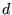. A more general and widely-used model is called the shadowing model .

The shadowing model consists of two parts. The first one is known as path loss model, which also predicts the mean received power at distance, denoted by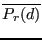. It uses a close-in distance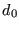as a reference.is computed relative to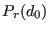as follows.(18.4)is called the path loss exponent, and is usually empirically determined by field measurement. From Eqn. (18.1) we know that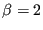for free space propagation. Table 18.1 gives some typical values of. Larger values correspond to more obstructions and hence faster decrease in average received power as distance becomes larger.can be computed from Eqn. (18.1).

Table 18.1: Some typical values of path loss exponentEnvironmentOutdoor Free space 2 Shadowed urban area 2.7 to 5 In building Line-of-sight 1.6 to 1.8 Obstructed 4 to 6

Table 18.2: Some typical values of shadowing deviation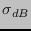Environment(dB) Outdoor 4 to 12 Office, hard partition 7 Office, soft partition 9.6 Factory, line-of-sight 3 to 6 Factory, obstructed 6.8

The path loss is usually measured in dB. So from Eqn. (18.4) we have(18.5)

The second part of the shadowing model reflects the variation of the received power at certain distance. It is a log-normal random variable, that is, it is of Gaussian distribution if measured in dB. The overall shadowing model is represented by(18.6)

whereis a Gaussian random variable with zero mean and standard deviation.is called the shadowing deviation, and is also obtained by measurement. Table  18.2 shows some typical values of. Eqn. (18.6) is also known as a log-normal shadowing model.

The shadowing model extends the ideal circle model to a richer statistic model: nodes can only probabilistically communicate when near the edge of the communication range.

Tom Henderson 2011-11-05